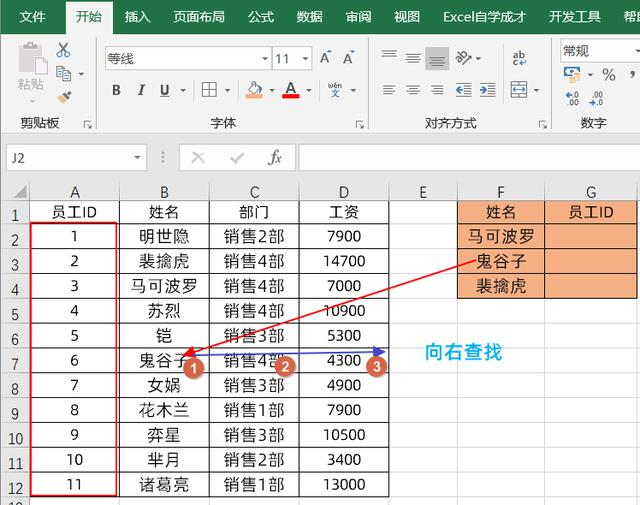=VLOOKUP(F2,A:B,2,0)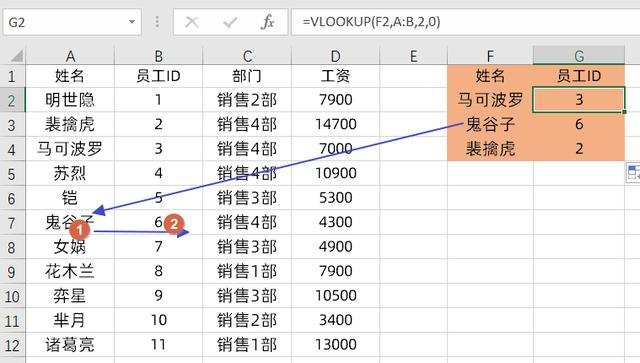### 方法一：使用if({1,0})方法

=VLOOKUP(F2,IF({1,0},B:B,A:A),2,0)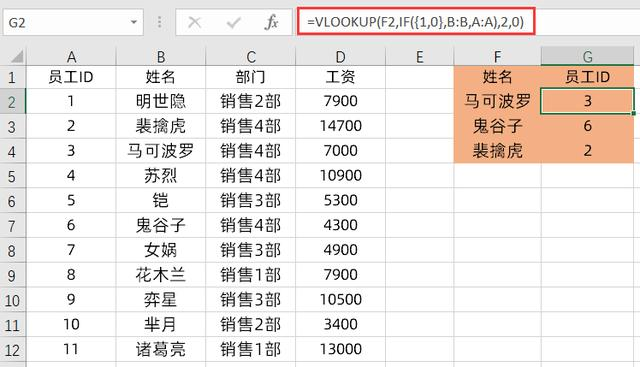=vlookup(查找值,if({1,0},查找列,结果列),2,0)

### 方法二：使用INDEX MATCH函数方法

=INDEX(A:A,MATCH(F2,B:B,0))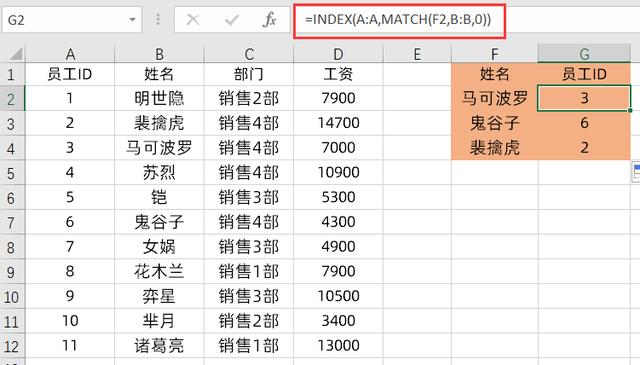=index(结果列,match(查找值,查找列,0))

### 方法三：使用Lookup函数方法

=LOOKUP(1,0/(B:B=F2),A:A)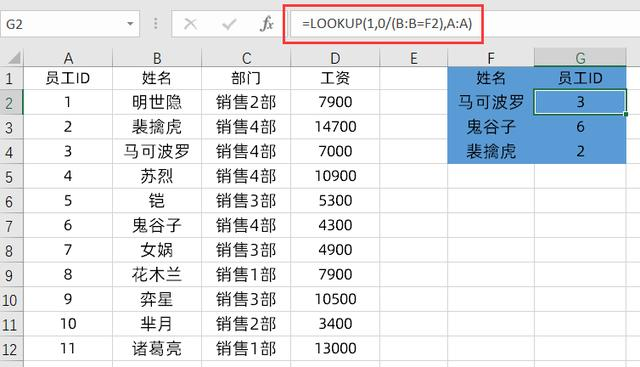=LOOKUP(1,0/(查找列=查找值),结果列)